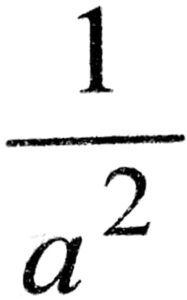4. CHEMICAL KINETICS

1. In chemical equation H2 (g) + I2 (g) ⇌ 2HI (g) the equilibrium constant Kp depends on

(A) total pressure
(B) catalyst used
(C) amount of H2 and I2
(D) temperature

2. If the rate of a reaction is expressed by, rate = k [A]2 [B], the order of reaction will be

(A) 2
(B) 3
(C) 1
(D) 0

3. In a reaction, 2X → Y, the concentration of X decreases from 0.50 M to 0.38 M in 10 min.What is the rate of reaction in M s-1 during this interval ?

(A) 2 x 10-4
(B) 4x 10-2
(C) 2 x 10-2
(D) 1x 10-2

4. Which of the following is an example of a fractional order reaction ?

(A) NH4NO2 → N2 + 2H2O
(B) NO + O3 NO2 + O2
(C) 2NO +Br2 → 2NOBr
(D) CH3CHO → CH4 + CO

5. The unit of rate constant for the reaction 2H2 + 2NO → 2H2O + N2 which has rate = K H2] [NO]2, is

(A) mol L-1s-1
(B) s-1
(C) mol-2 L2 5-1
(D) mol L-1

6. The rate constant of a reaction depends upon

(A) temperature of the reaction
(B) extent of the reaction
(C) initial concentration of the reactants
(D) the time of completion of reaction

7. The chemical reaction, 203 → 302 proceeds as

O3 ⇌  O2 + [O] (fast)
[O] + O3 → 2O2 (slow)
The rate law expression will be

(A) Rate = k [O] [O3]
(B) Rate = k [O3]2 [O2]-1
(C) Rate = k [O3]2
(D) Rate = k [O2] [O]

8. The unit of rate and rate constant are same for a

(A) zero order reaction
(B) first order reaction
(C) second order reaction
(D) third order reaction

9. For a unimolecular reaction

(A) the order and molecularity of the slowest step are equal to one
(B) molecularity of the reaction can be zero, one or two
(C) molecularity of the reaction can be determined only experimentally
(D) more than one reacting species are involved in one step

10. The order of reaction is decided by

(A) temperature
(B) mechanism of reaction as well as relative concentration of reactants
(C) molecularity
(D) pressure

11. Radioactive disintegration is an example of

(A) zero order reaction
(B) first order reaction
(C) second order reaction
(D) third order reaction

12. The hydrolysis of ethyl acetate :

CH3COOC2H5 + H2O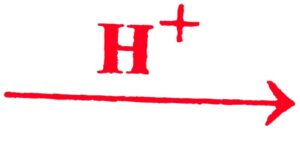CH3COOH + C2H5OH is a reaction of

(A) zero order
(B) pseudo first order
(C) second order
(D) third order

13. Rate of which reaction increases with temperature ?

(A) Exothermic reaction
(B) Endothermic reaction
(C) (A) and (B) both
(D) None of the above

14. The activation energy in a chemical reaction is defined as :

(A) the difference in energies of reactants and products
(B) the sum of energies of reactants and products
(C) the difference in energy of intermediate complex with the average energy of reactants and products
(D) the difference in energy of intermediate complex and the average energy of reactants

15. The temperature dependence of the rate of a chemical reaction can be explained by Arrhenius equation which is

(A) k = Ae Ea/RT
(B) k = Ae -Ea/RT
(C) k = Ae x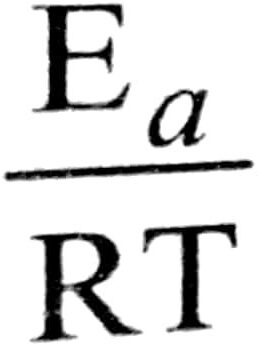(D) k = Ae x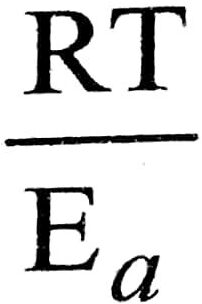16. The role of a catalyst is to change

(A) Gibbs energy of reaction
(B) enthalpy of reaction
(C) activation energy of reaction
(D) equilibrium constant

17. In the presence of a catalyst, the heat evolved or absorbed during the reaction

(A) increases
(B) decreases
(C) may increase or decrease
(D) remains unchanged

18. Rate law for the reaction A + B → C is found to be Rate = k [A] [B] Concentration of reactant ‘B’ is doubled, keeping the concentration of ‘A’ constant, the value of rate constant will be

(A) the same
(B) doubled
(D) halved

19. The value of rate constant of a pseudo first order reaction

(A) depends on the concentration of reactants present in small amount
(B) depends on the concentration of reactants presents in excess
(C) is independent of the concentration of reactants
(D) depends only on temperature

20. t1/2 for first order reaction is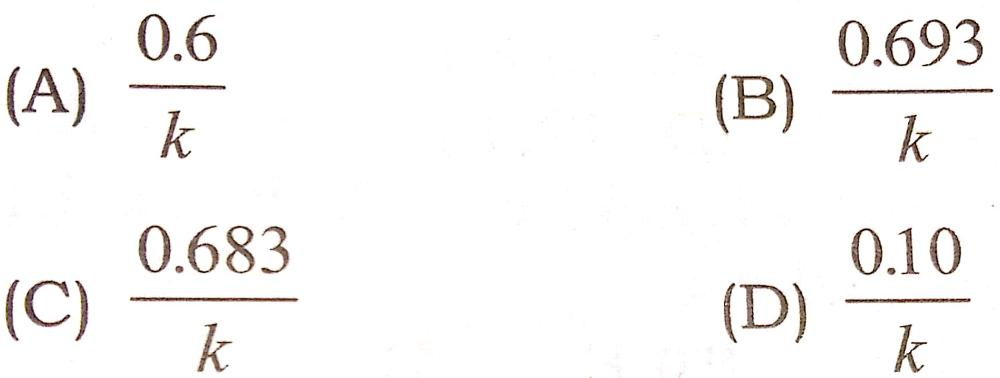21. The rate at which a substance reacts, depends upon its

(A) Atomic mass
(B) Equivalent mass
(C) Molecular mass
(D) Active mass

22. For a zero order reaction

(A) t1/2(B) t1/2 ∝ 1/a
(C) t1/2a2
(D) t1/2